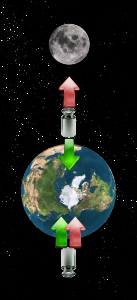# Moon's Gravity Engine

Sat, 09/22/2012 - 23:30

### Themes:

Being working in the renewable energy field since some years, sometimes unlikely ideas about some "free" power generation systems came to my mind.

The basic concept of a Moon's Gravity Engine, roughly simplifying,  is this:

• Moon is apparently rotating around Earth a bit slowly than one turn per day.
• So if at time $T_0$ I have the Moon over me, at time $T_0+12h$ it is at the opposite side of Earth.
• Moon gravity produce some effect also here at the Earth
• While Moon is over me  the weight of a generic mass near me will be reduced.
• While Moon is at the opposite side the weight of a generic mass near me will be larger.
• Than if I lift a mass when Moon is over me and I lower it (recovering the potential energy) when Moon is at the opposite side than I should have a net energy available for each cycle.
• That's an engine: a Moon's Gravity Engine!

This could sound like a crazy idea but why not to try to do some calculations to see how much (or little) crazy it is?

## Model descriptionWe're going to keep things simple:

• Moon and Earth are assumed to be spheres with homogeneous mass distribution.
• Moon's orbit is assumed to lay on the Earth's equatorial plane.
• Moon's orbit is assumed to be a circle centered on the Earth center.
• Sun and other bodies influence is not considered.
• Moon's Gravity Engine operating at an equatorial location.
• Newtonian's mechanics is valid.

In other words what are we missing?

• Earth is a geoid and moon id a moonoid...
• Moon's orbit is on a plane that is about $5°$ skewed with respect to the ecliptic that is itself about $23°$ skewed with respect to the Earth's equatorial plane
• Moon's orbit is obviously an ellipse and the Earth-Moon distance varies about $\pm5.5\%$ with respect to its the mean value.
• Earth-Moon system does not move in a completely empty space so is actually influenced by other celestial bodies. The most important effect we're going to ignore is that the Earth-Moon center of gravity is not moving in a straight line but it is actually revolving around the Sun (and also with it around the center of our galaxy and so on).
• Albert told us that Newton was wrong... ...but we're going to look at the half full glass!